# User:Nikita2/sandbox

## Contents

#### $\mathcal N$-property of a function $f$, continuous on an interval $[a,b]$

For any set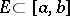of measure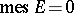, the image of this set,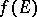, also has measure zero. It was introduced by N.N. Luzin in 1915 (see ). The following assertions hold.

1) A function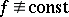onsuch that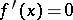almost-everywhere ondoes not have the Luzin-property.

2) Ifdoes not have the Luzin-property, then onthere is a perfect setof measure zero such that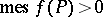.

3) An absolutely continuous function has the Luzin-property.

4) Ifhas the Luzin-property and has bounded variation on(as well as being continuous on), thenis absolutely continuous on(the Banach–Zaretskii theorem).

5) Ifdoes not decrease onand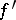is finite on, thenhas the Luzin-property.

6) In order thatbe measurable for every measurable setit is necessary and sufficient thathave the Luzin-property on.

7) A functionthat has the Luzin-property has a derivativeon the set for which any non-empty portion of it has positive measure.

8) For any perfect nowhere-dense set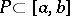there is a functionhaving the Luzin-property onand such thatdoes not exist at any point of.

The concept of Luzin's-property can be generalized to functions of several variables and functions of a more general nature, defined on measure spaces.